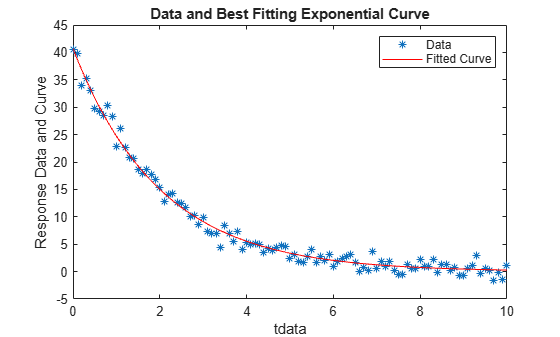# Curve Fitting via Optimization

This example shows how to fit a nonlinear function to data. For this example, the nonlinear function is the standard exponential decay curve

`$y\left(t\right)=A\mathrm{exp}\left(-\lambda t\right),$`

where $y\left(t\right)$ is the response at time $t$, and $A$ and $\lambda$ are the parameters to fit. Fitting the curve means finding parameters $A$ and $\lambda$ that minimize the sum of squared errors

`$\sum _{i=1}^{n}{\left({y}_{i}-A\mathrm{exp}\left(-\lambda {t}_{i}\right)\right)}^{2},$`

where the times are ${t}_{i}$ and the responses are ${y}_{i},i=1,\dots ,n$. The sum of squared errors is the objective function.

### Create Sample Data

Usually, you have data from measurements. For this example, create artificial data based on a model with $A=40$ and $\lambda =0.5$, with normally distributed pseudorandom errors.

```rng default % for reproducibility tdata = 0:0.1:10; ydata = 40*exp(-0.5*tdata) + randn(size(tdata));```

### Write Objective Function

Write a function that accepts parameters `A` and `lambda` and data `tdata` and `ydata`, and returns the sum of squared errors for the model $y\left(t\right)$. Put all the variables to optimize (`A` and `lambda`) in a single vector variable (`x`). For more information, see Minimizing Functions of Several Variables.

`type sseval`
```function sse = sseval(x,tdata,ydata) A = x(1); lambda = x(2); sse = sum((ydata - A*exp(-lambda*tdata)).^2); ```

Save this objective function as a file named `sseval.m` on your MATLAB® path.

The `fminsearch` solver applies to functions of one variable, `x`. However, the `sseval` function has three variables. The extra variables `tdata` and `ydata` are not variables to optimize, but are data for the optimization. Define the objective function for `fminsearch` as a function of `x` alone:

`fun = @(x)sseval(x,tdata,ydata);`

For information about including extra parameters such as `tdata` and `ydata`, see Parameterizing Functions.

### Find the Best Fitting Parameters

Start from a random positive set of parameters `x0`, and have `fminsearch` find the parameters that minimize the objective function.

```x0 = rand(2,1); bestx = fminsearch(fun,x0)```
```bestx = 2×1 40.6877 0.4984 ```

The result `bestx` is reasonably near the parameters that generated the data, `A = 40` and `lambda = 0.5`.

### Check the Fit Quality

To check the quality of the fit, plot the data and the resulting fitted response curve. Create the response curve from the returned parameters of your model.

```A = bestx(1); lambda = bestx(2); yfit = A*exp(-lambda*tdata); plot(tdata,ydata,'*'); hold on plot(tdata,yfit,'r'); xlabel('tdata') ylabel('Response Data and Curve') title('Data and Best Fitting Exponential Curve') legend('Data','Fitted Curve') hold off```